# Maharashtra Board 10th Class Maths Part 1 Practice Set 1.2 Solutions Chapter 1 Linear Equations in Two Variables

Balbharti Maharashtra State Board Class 10 Maths Solutions covers the Practice Set 1.2 Algebra 10th Class Maths Part 1 Answers Solutions Chapter 1 Linear Equations in Two Variables.

## Practice Set 1.2 Algebra 10th Std Maths Part 1 Answers Chapter 1 Linear Equations in Two Variables

10th Maths 2 Practice Set 1.2 Question 1.
Complete the following table to draw graph of the equations.
i. x + y = 3
ii. x – y = 4
i. x + y = 3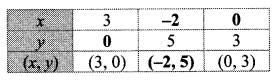ii. x – y = 4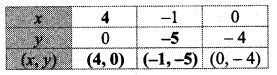Linear Equations In Two Variables Practice Set 1.2  Question 2.
Solve the following simultaneous equations graphically.
i. x + y = 6 ; x – y = 4
ii. x + y = 5 ; x – y = 3
iii. x + y = 0 ; 2x – y = 9
iv. 3x – y = 2 ; 2x – y = 3
v. 3x – 4y = -7 ; 5x – 2y = 0
vi. 2x – 3y = 4 ; 3y – x = 4
Solution:
i. The given simultaneous equations are
x + y = 6                                                                                                        x – y = 4
∴ y = 6 – x                                                                                                     ∴ y = x – 4The two lines intersect at point (5, 1).
∴ x = 5 and y = 1 is the solution of the simultaneous equations x + y = 6 and x – y = 4.

ii. The given simultaneous equations are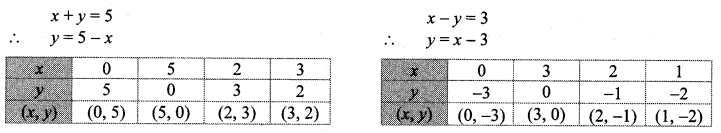The two lines intersect at point (4, 1).
∴ x = 4 and y = 1 is the solution of the simultaneous equations x+y = 5 and x – y = 3.

iii. The given simultaneous equations are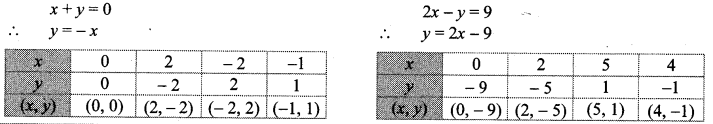The two lines intersect at point (3, -3).
∴ x = 3 and y = -3 is the solution of the simultaneous equations x + y = 0 and 2x – y = 9.

iv. The given simultaneous equations are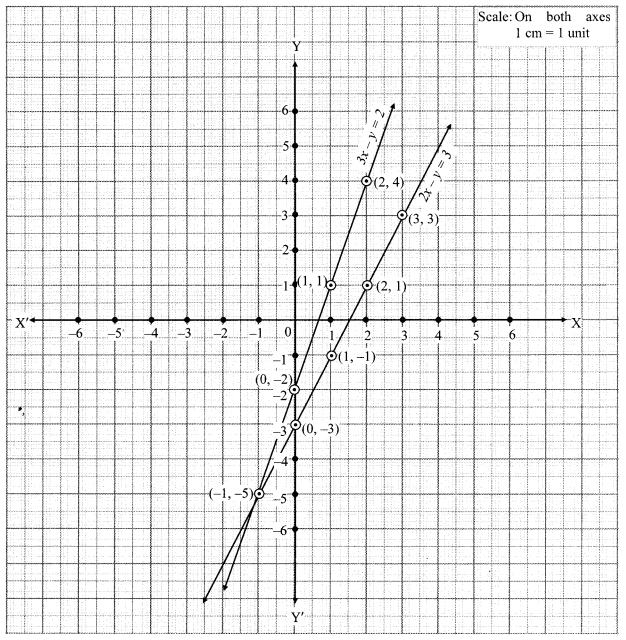The two lines intersect at point (-1, -5).
∴ x = -1 and y = -5 is the solution of the simultaneous equations 3x- y = 2 and 2x- y = 3.

v. The given simultaneous equations are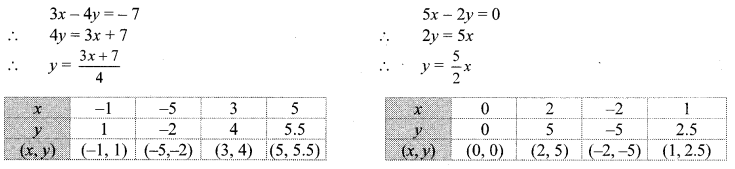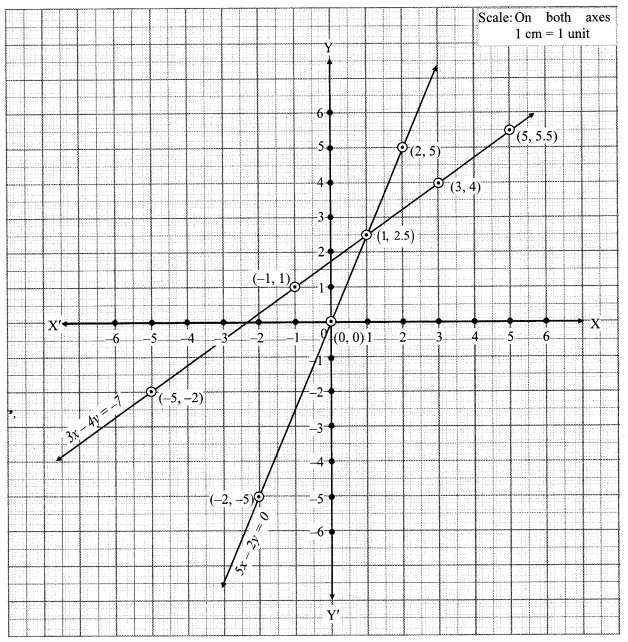The two lines intersect at point (1, 2.5).
∴ x = 1 and y = 2.5 is the solution of the simultaneous equations 3x – 4y = -7 and 5x – 2y = 0.

vi. The given simultaneous equations are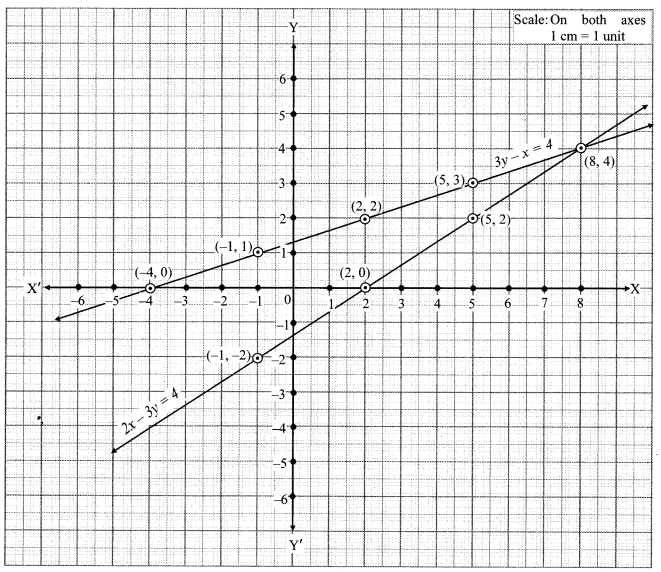The two lines intersect at point (8, 4).
∴ x = 8 and y = 4 is the solution of the simultaneous equations 2x – 3y = 4 and 3y – x = 4.

10th Math Part 2 Practice Set 1.2  Question 1.
Solve the following simultaneous equations by graphical method. Complete the following tables to get ordered pairs.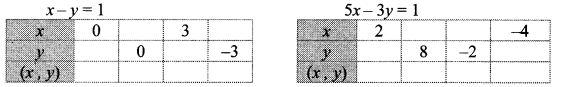i. Plot the above ordered pairs on the same co-ordinate plane.
ii. Draw graphs of the equations.
iii. Note the co-ordinates of the point of intersection of the two graphs. Write solution of these equations. (Textbook pg. no. 8)
Solution: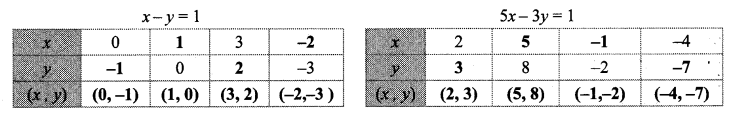The two lines intersect at point (-1, -2).
∴ (x , y) = (-1, -2) is the solution of the given simultaneous equations.

Mathematics Part 1 Standard 9 Practice Set 1.2 Answer  Question 1.
Solve the above equations by method of elimination. Check your solution with the solution obtained by graphical method. (Textbook pg. no. 8)
Solution:
The given simultaneous equations are
x – y = 1 …(i)
5x – 3y = 1 …(ii)
Multiplying equation (i) by 3, we get
3x – 3y = 3 …(iii)
Subtracting equation (iii) from (ii), we get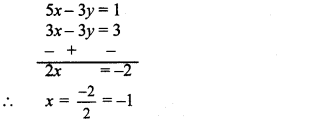Substituting x = -1 in equation (i), we get
-1 -y= 1
∴ -y = 1 + 1
∴ -y = 2
∴ y = -2
∴ (x,y) = (-1, -2) is the solution of the given simultaneous equations.
∴ The solution obtained by elimination method and by graphical method is the same.

1.2 Maths Class 10 Question 2.
The following table contains the values of x and y co-ordinates for ordered pairs to draw the graph of 5x – 3y = 1.i. Is it easy to plot these points?
ii. Which precaution is to be taken to find ordered pairs so that plotting of points becomes easy? (Textbook pg. no. 8)
Solution:
i. No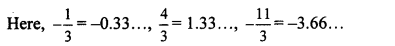The above numbers are non-terminating and recurring decimals.
∴ It is not easy to plot the given points.

ii. While finding ordered pairs, numbers should be selected in such a way that the co-ordinates obtained will be integers.

Linear Equations ¡n Two Variables Class 10 Maths Question 3.
To solve simultaneous equations x + 2y = 4; 3x + 6y = 12 graphically, following are the ordered pairs.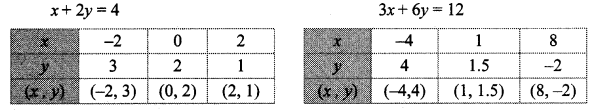Plotting the above ordered pairs, graph is drawn. Observe it and find answers of the following questions.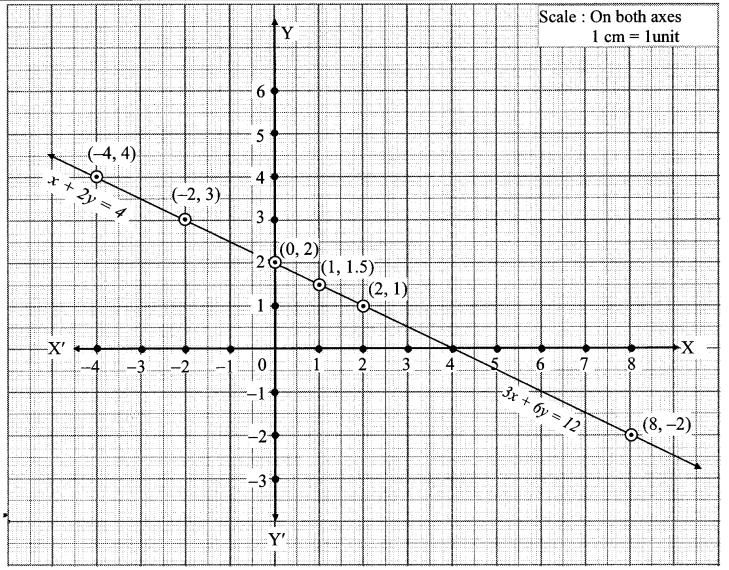i. Are the graphs of both the equations different or same?
ii. What are the solutions of the two equations x + 2y = 4 and 3x + 6y = 12? How many solutions are possible?
iii. What are the relations between coefficients of x, coefficients of y and constant terms in both the equations?
iv. What conclusion can you draw when two equations are given but the graph is only one line? (Textbook pg. no. 9)
Solution:
i. The graphs of both the equations are same.
ii. The solutions of the given equations are (-2, 3), (0, 2), (1, 1.5), etc.
∴ Infinite solutions are possible.
iii. Ratio of coefficients of x = $$\frac { 1 }{ 3 }$$
Ratio of coefficients of y = $$\frac { 2 }{ 6 }$$ = $$\frac { 1 }{ 3 }$$
Ratio of constant terms = $$\frac { 4 }{ 12 }$$ = $$\frac { 1 }{ 3 }$$
∴ Ratios of coefficients of x = ratio of coefficients of y = ratio of the constant terms
iv. When two equations are given but the graph is only one line, the equations will have infinite solutions.

Class 10 Maths Part 1 Practice Set 1.2 Question 4.
Draw graphs of x- 2y = 4, 2x – 4y = 12 on the same co-ordinate plane. Observe it. Think of the relation between the coefficients of x, coefficients ofy and the constant terms and draw the inference. (Textbook pg. no. 10)
Solution: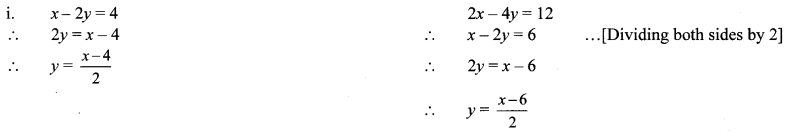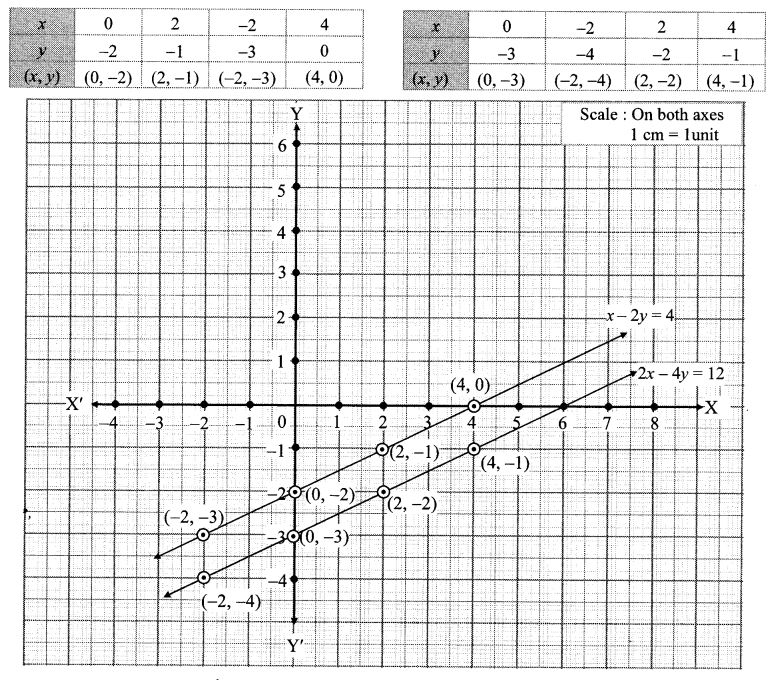ii. Ratio of coefficients of x =$$\frac { 1 }{ 2 }$$
Ratio of coefficients of y = $$\frac { -2 }{ -4 }$$ = $$\frac { 1 }{ 2 }$$
Ratio of constant terms = $$\frac { 4 }{ 12 }$$ = $$\frac { 1 }{ 3 }$$
∴ Ratio of coefficients of x = ratio of coefficients of y ratio of constant terms
iii. If ratio of coefficients of x = ratio of coefficients of y ≠ ratio of constant terms, then the graphs of the two equations will be parallel to each other.

Condition of consistency in Equations: